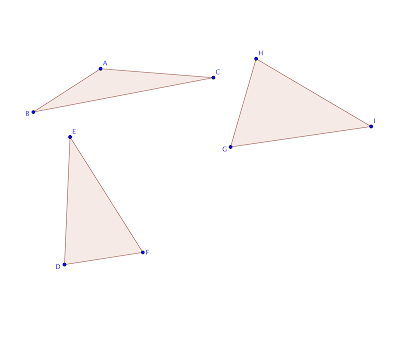## Monday, November 18, 2013

### "Eccentricity" of a triangle

How do you measure the "eccentricity" of a shape? If one examines a triangle, how deformed is it compared to an equilateral triangle?

Lots of ideas suggest themselves, but the simplest suggests comparing a two-dimensional to a one-dimensional property: area to perimeter.

Specifically, there are three possible formulations that come to mind:

1. $\frac{perimete{r}_{EQ▵}}{perimete{r}_{▵}}$, where the equilateral and deformed triangles have equal areas.

2. $\frac{are{a}_{▵}}{are{a}_{EQ▵}}$, where the equilateral and deformed triangles have equal perimeters.

3. $\frac{perimeter}{\sqrt{area}}$

This ratio can be calculated for both triangles; the equilateral triangle has the lowest possible ratio for a triangle, so the closer a triangle's ratio is to the equilateral triangle's, the less deformed it is.I created three triangles and ranked them using each formula. All three formulas yielded the same result: triangle ABC was the most deformed, followed by triangle EDF; triangle GHI was the least deformed. This accords visually.

Given that all three formulas work, I would use the latter, since it is the simplest. Knowledge of an equilateral triangle's ratio is unnecessary. In fact, this formula can be used to compare the deformity of two quadrilaterals, two pentagons, etc. It was the formula I used to put a statistic to how deformed gerrymandered congressional districts were.